# ISEE Upper Level Quantitative : How to find the length of the side of a trapezoid

## Example Questions

### Example Question #1 : How To Find The Length Of The Side Of A Trapezoid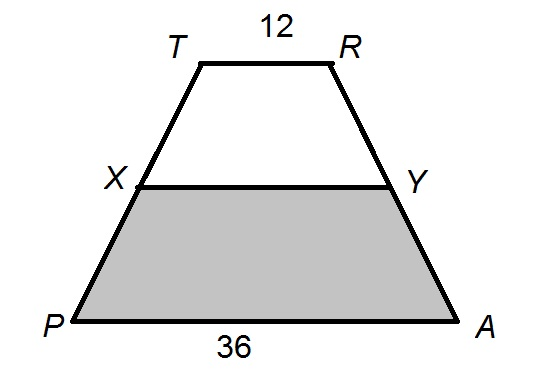In the above diagram, which depicts Trapezoid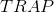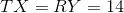and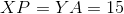. Which is the greater quantity?

(a)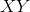(b) 24

It is impossible to determine which is greater from the information given

(a) is the greater quantity

(a) and (b) are equal

(b) is the greater quantity

(b) is the greater quantity

Explanation:

To see that (b) is the greater quantity of the two, it suffices to construct the midsegment of the trapezoid - the segment which has as its endpoints the midpoints of legs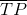and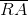. Since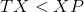and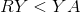, the midsegment,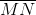, is positioned as follows: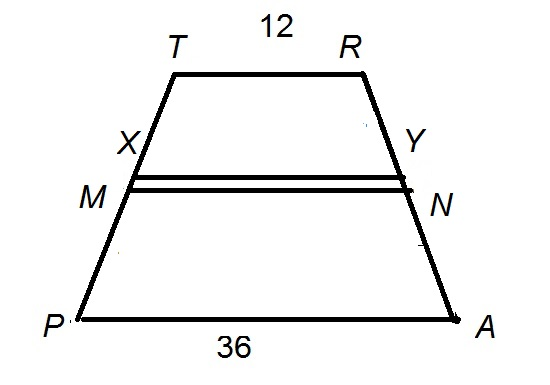The length of the midsegment is half the sum of the bases, so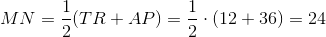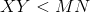, so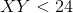.

### Example Question #2 : How To Find The Length Of The Side Of A Trapezoid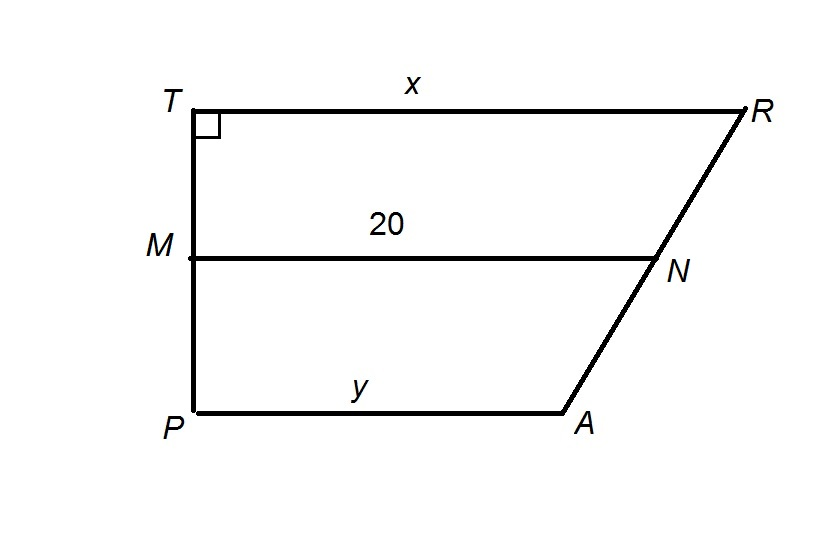Figure NOT drawn to scale.

The above figure depicts Trapezoidwith midsegment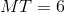, and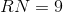.

Give the area of Trapezoid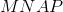in terms of.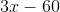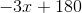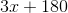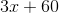Explanation:

The midsegment of a trapezoid has as its length half the sum of the lengths of the bases, which here are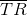and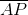: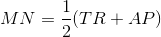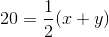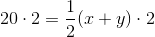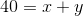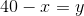Therefore,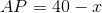The area of Trapezoidis one half multiplied by the height,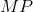, multiplied by the sum of the lengths of the bases,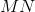and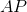. The midsegment of a trapezoid bisects both legs, so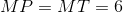, and the area is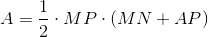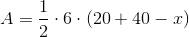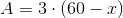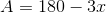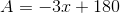### Example Question #3 : How To Find The Length Of The Side Of A TrapezoidThe above figure depicts Trapezoidwith midsegment. Expressin terms of.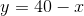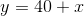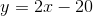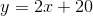Explanation:

The midsegment of a trapezoid has as its length half the sum of the lengths of the bases, which here areand: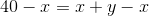The correct choice is.